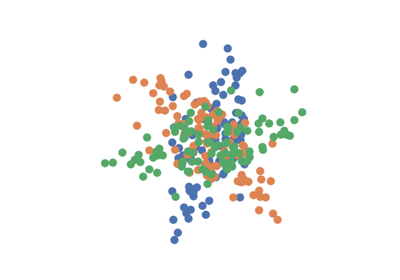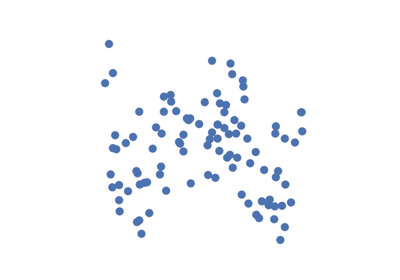# perm_test¶

hyppo.tools.perm_test(calc_stat, x, y, reps=1000, workers=1, is_distsim=True, perm_blocks=None, random_state=None)

Permutation test for the p-value of a nonparametric test.

This process is completed by first randomly permuting $$y$$ to estimate the null distribution and then calculating the probability of observing a test statistic, under the null, at least as extreme as the observed test statistic.

Parameters
• calc_stat (callable) -- The method used to calculate the test statistic (must use hyppo API).

• x,y (ndarray) -- Input data matrices. x and y must have the same number of samples. That is, the shapes must be (n, p) and (n, q) where n is the number of samples and p and q are the number of dimensions. Alternatively, x and y can be distance or similarity matrices, where the shapes must both be (n, n).

• reps (int, default: 1000) -- The number of replications used to estimate the null distribution when using the permutation test used to calculate the p-value.

• workers (int, default: 1) -- The number of cores to parallelize the p-value computation over. Supply -1 to use all cores available to the Process.

• is_distsim (bool, default: True) -- Whether or not x and y are distance or similarity matrices.

• perm_blocks (ndarray, default: None) -- Defines blocks of exchangeable samples during the permutation test. If None, all samples can be permuted with one another. Requires n rows. Constructs a tree graph with all samples initially at the root node. Each column partitions samples from the same leaf with shared column label into a child of that leaf. During the permutation test, samples within the same final leaf node are exchangeable and blocks of samples with a common parent node are exchangeable. If a column value is negative, the resulting block is unexchangeable.

Returns

## Examples using hyppo.tools.perm_test¶